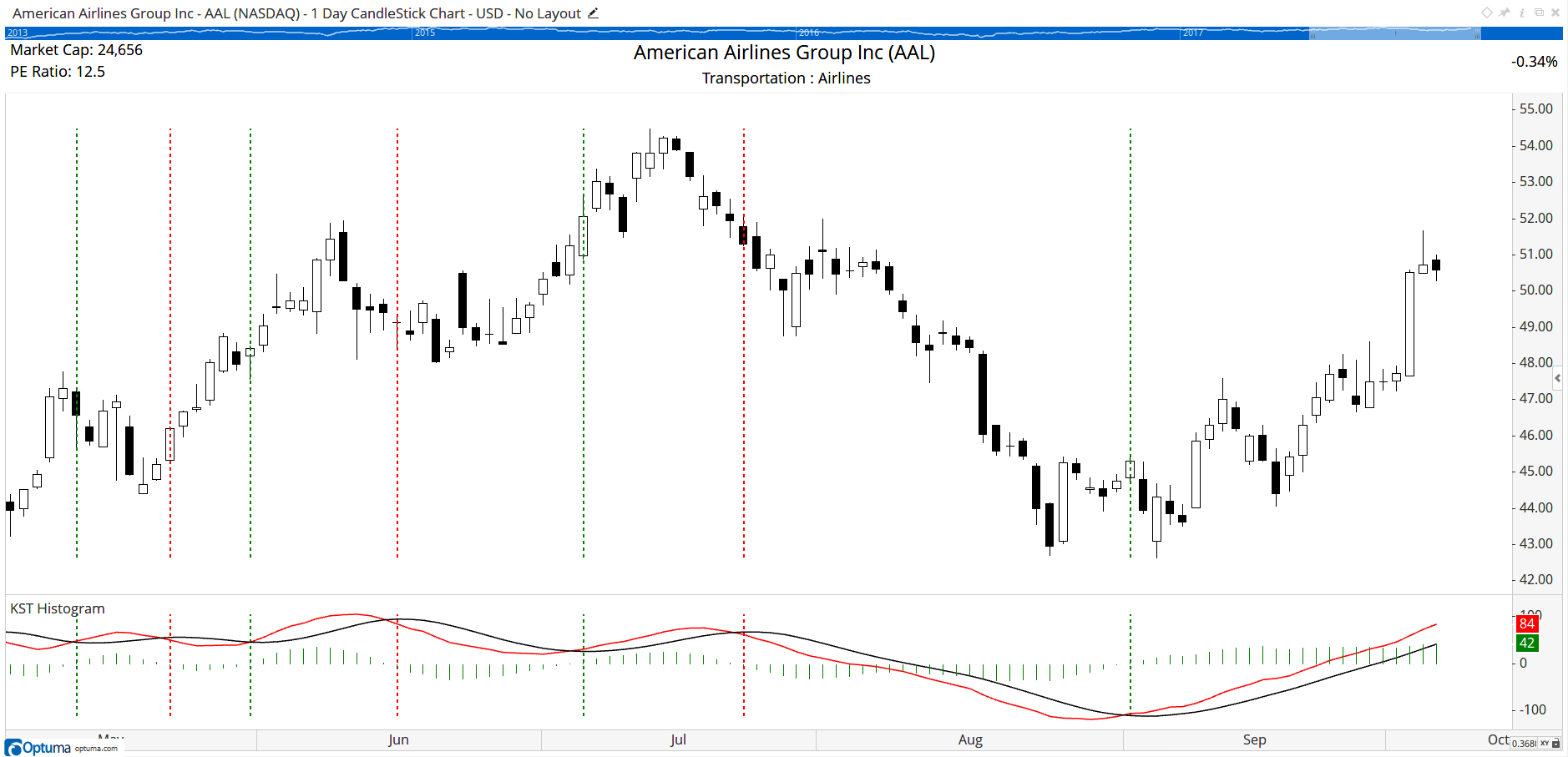Select Page

# Reply To: Pring's KST Indicator

Optuma Forums Optuma Scripting Pring's KST Indicator Reply To: Pring's KST Indicator

#41659

To find when the KST crosses the signal line use the following in a Show Bar or scan formula.

Cross Above:

//Create the Rates of Change
r24 = MA(ROC(BARS=24), BARS=9, CALC=Close) * 4;
r18 = MA(ROC(BARS=18), BARS=9, CALC=Close) * 3;
r12 = MA(ROC(BARS=12), BARS=6, CALC=Close) * 2;
r9 = MA(ROC(BARS=9), BARS=6, CALC=Close);

KST1 = r24 + r18 + r12 + r9;
//Signal Line
KSTMA = MA(KST1, BARS=9, CALC=Close);
KST1 CrossesAbove KSTMA

Cross Below:

//Create the Rates of Change
r24 = MA(ROC(BARS=24), BARS=9, CALC=Close) * 4;
r18 = MA(ROC(BARS=18), BARS=9, CALC=Close) * 3;
r12 = MA(ROC(BARS=12), BARS=6, CALC=Close) * 2;
r9 = MA(ROC(BARS=9), BARS=6, CALC=Close);
KST1 = r24 + r18 + r12 + r9;
//Signal Line
KSTMA = MA(KST1, BARS=9, CALC=Close);
KST1 CrossesBelow KSTMA

In this example the Show Bar has been set to Lines instead of Arrows: